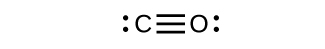# 4.3.1: Valence Bond Theory and Hybrid Orbitals (Problems)

$$\newcommand{\vecs}{\overset { \rightharpoonup} {\mathbf{#1}} }$$ $$\newcommand{\vecd}{\overset{-\!-\!\rightharpoonup}{\vphantom{a}\smash {#1}}}$$$$\newcommand{\id}{\mathrm{id}}$$ $$\newcommand{\Span}{\mathrm{span}}$$ $$\newcommand{\kernel}{\mathrm{null}\,}$$ $$\newcommand{\range}{\mathrm{range}\,}$$ $$\newcommand{\RealPart}{\mathrm{Re}}$$ $$\newcommand{\ImaginaryPart}{\mathrm{Im}}$$ $$\newcommand{\Argument}{\mathrm{Arg}}$$ $$\newcommand{\norm}{\| #1 \|}$$ $$\newcommand{\inner}{\langle #1, #2 \rangle}$$ $$\newcommand{\Span}{\mathrm{span}}$$ $$\newcommand{\id}{\mathrm{id}}$$ $$\newcommand{\Span}{\mathrm{span}}$$ $$\newcommand{\kernel}{\mathrm{null}\,}$$ $$\newcommand{\range}{\mathrm{range}\,}$$ $$\newcommand{\RealPart}{\mathrm{Re}}$$ $$\newcommand{\ImaginaryPart}{\mathrm{Im}}$$ $$\newcommand{\Argument}{\mathrm{Arg}}$$ $$\newcommand{\norm}{\| #1 \|}$$ $$\newcommand{\inner}{\langle #1, #2 \rangle}$$ $$\newcommand{\Span}{\mathrm{span}}$$

PROBLEM $$\PageIndex{1}$$

Explain how σ and π bonds are similar and how they are different.

Similarities: Both types of bonds result from overlap of atomic orbitals on adjacent atoms and contain a maximum of two electrons. Differences: σ bonds are stronger and result from end-to-end overlap and all single bonds are σ bonds; π bonds between the same two atoms are weaker because they result from side-by-side overlap, and multiple bonds contain one or more π bonds (in addition to a σ bond).

PROBLEM $$\PageIndex{2}$$

How many σ and π bonds are present in the molecule HCN?

$$\ce{H–C≡N}$$ has two σ (H–C and C–N) and two π (making the CN triple bond).PROBLEM $$\PageIndex{3}$$

A friend tells you N2 has three π bonds due to overlap of the three p-orbitals on each N atom. Do you agree?

No, two of the p orbitals (one on each N) will be oriented end-to-end and will form a σ bond.PROBLEM $$\PageIndex{4}$$

Draw the Lewis structures for CO2 and CO, and predict the number of σ and π bonds for each molecule.
a. CO2
b. CO

2 σ 2 π1 σ 2 πPROBLEM $$\PageIndex{5}$$

Why is the concept of hybridization required in valence bond theory?

Hybridization is introduced to explain the geometry of bonding orbitals in valance bond theory.

PROBLEM $$\PageIndex{6}$$

Give the shape that describes each hybrid orbital set:

(a) sp2

(b) spPROBLEM $$\PageIndex{7}$$

What is the hybridization of the central atom in each of the following?

(a) BeH2

(b) $$\ce{PO4^3-}$$

linear

tetrahedral

PROBLEM $$\PageIndex{8}$$

A molecule with the formula AB3 could have one of two different shapes. Give the shape and the hybridization of the central A atom for each.

trigonal planar, sp2; trigonal pyramidal (one lone pair on A) sp3

PROBLEM $$\PageIndex{9}$$

Methionine, CH3SCH2CH2CH(NH2)CO2H, is an amino acid found in proteins. Based on the Lewis structure of this compound, what is the hybridization type of each carbon, oxygen, the nitrogen, and the sulfur?PROBLEM $$\PageIndex{10}$$

Two important industrial chemicals, ethene, C2H4, and propene, C3H6, are produced by the steam (or thermal) cracking process:

$$\ce{2C3H8}(g)⟶\ce{C2H4}(g)+\ce{C3H6}(g)+\ce{CH4}(g)+\ce{H2}(g)$$

For each of the four carbon compounds, do the following:

(a) Draw a Lewis structure.

(b) Predict the geometry about the carbon atom.

(c) Determine the hybridization of each type of carbon atom.C3H8: tetrahedral

C2H4: trigonal planar

C3H6: trigonal planar (1&2) and tetrahedral (3)

CH4: tetrahedralPROBLEM $$\PageIndex{11}$$

Consider nitrous acid, HNO2 (HONO).

(a) Write a Lewis structure.

(b) What are the electron pair and molecular geometries of the internal oxygen and nitrogen atoms in the HNO2 molecule?

(c) What is the hybridization on the internal oxygen and nitrogen atoms in HNO2?Electron pair: O: tetrahedral, N: trigonal planar

Molecular geometry: O: bent (109), N: trigonal planar

O: sp3

N: sp2

PROBLEM $$\PageIndex{12}$$

Identify the hybridization of each carbon atom in the following molecule. (The arrangement of atoms is given; you need to determine how many bonds connect each pair of atoms.)